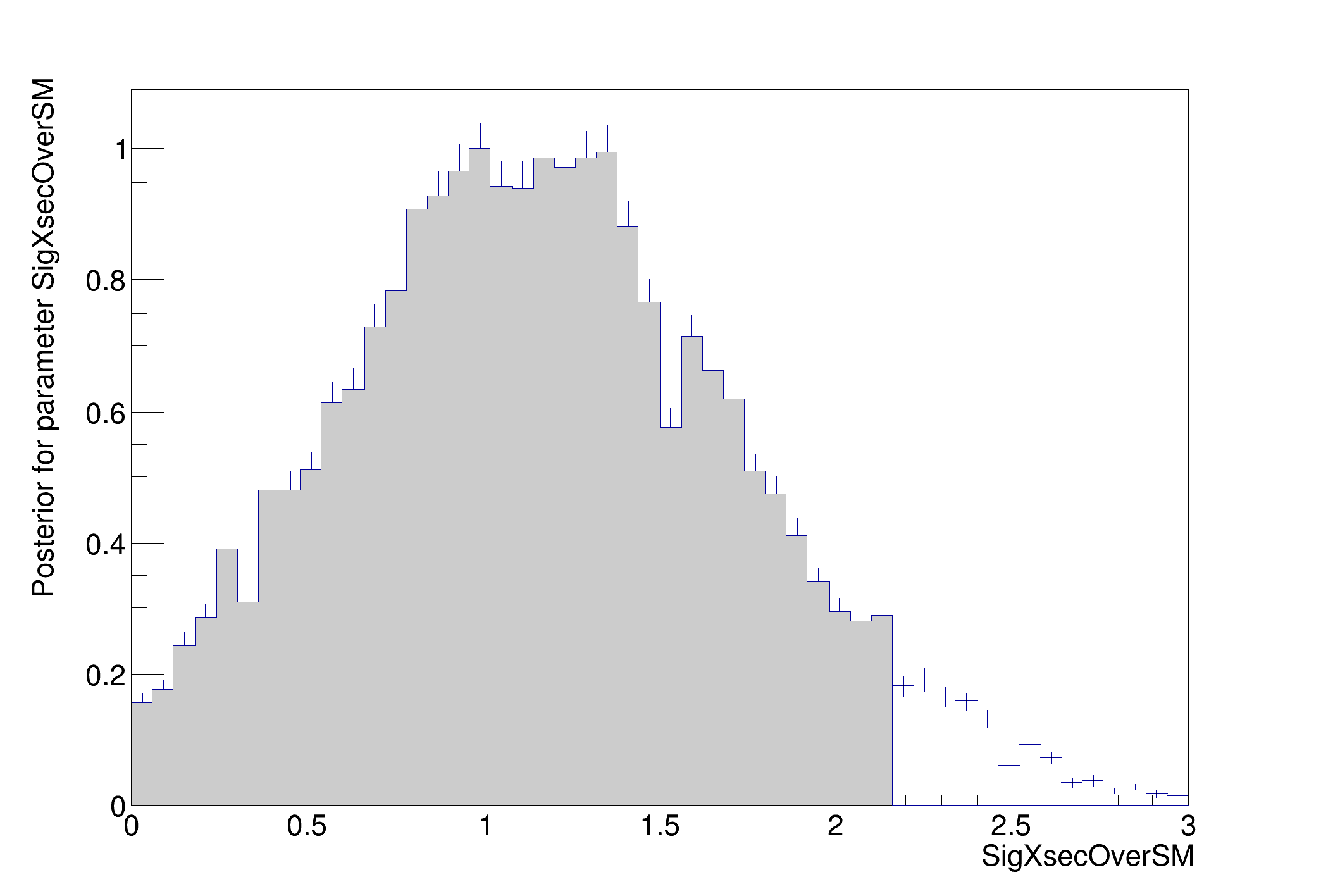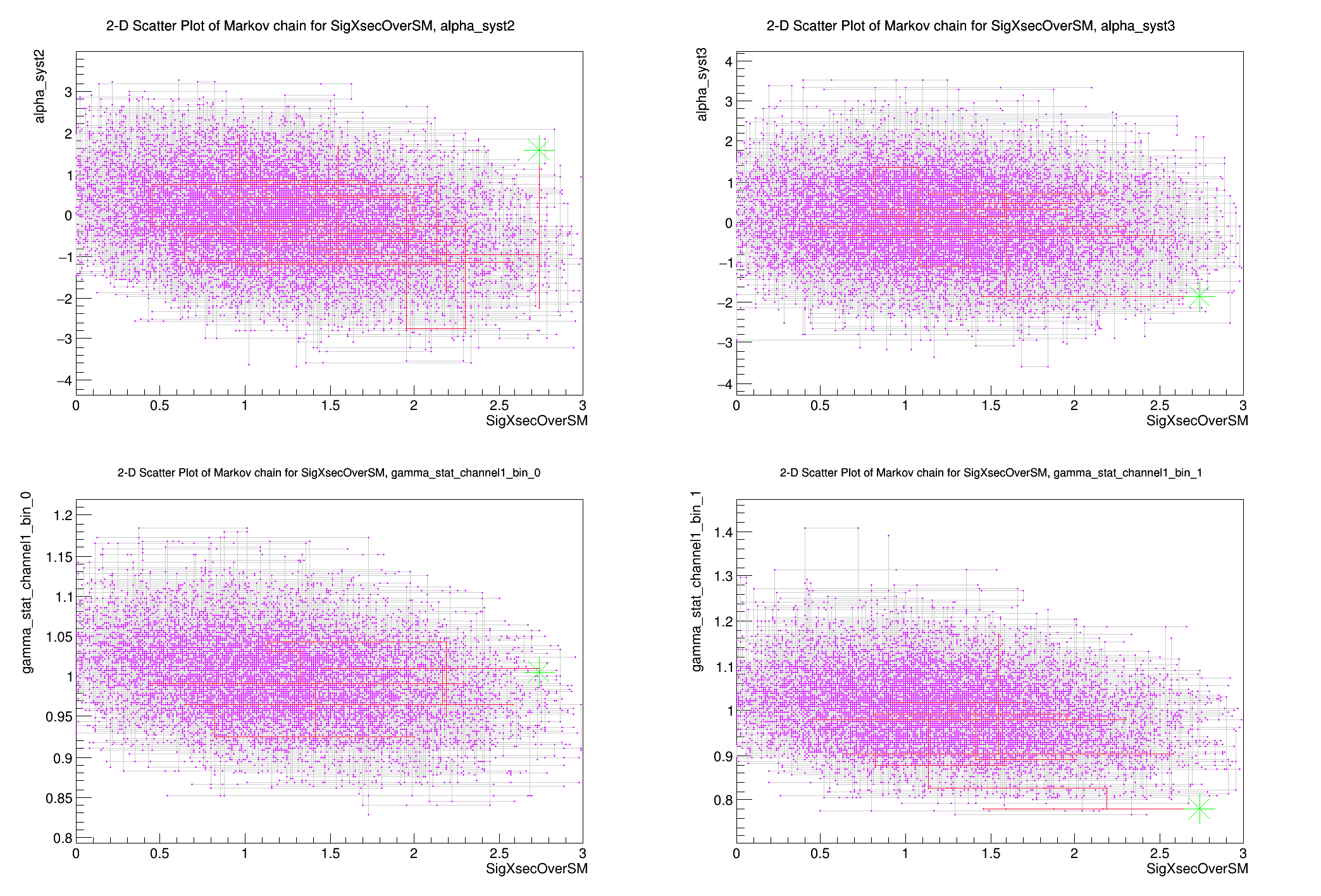ROOT   Reference GuideStandardBayesianMCMCDemo.C File Reference

## Detailed DescriptionStandard demo of the Bayesian MCMC calculator

This is a standard demo that can be used with any ROOT file prepared in the standard way. You specify:

• name for input ROOT file
• name of workspace inside ROOT file that holds model and data
• name of ModelConfig that specifies details for calculator tools
• name of dataset

With default parameters the macro will attempt to run the standard hist2workspace example and read the ROOT file that it produces.

The actual heart of the demo is only about 10 lines long.

The MCMCCalculator is a Bayesian tool that uses the Metropolis-Hastings algorithm to efficiently integrate in many dimensions. It is not as accurate as the BayesianCalculator for simple problems, but it scales to much more complicated cases.␛[1mRooFit v3.60 -- Developed by Wouter Verkerke and David Kirkby␛[0m
Copyright (C) 2000-2013 NIKHEF, University of California & Stanford University
[#1] INFO:Minization -- p.d.f. provides expected number of events, including extended term in likelihood.
[#1] INFO:Minization -- createNLL: caching constraint set under name CONSTR_OF_PDF_simPdf_FOR_OBS_channelCat:obs_x_channel1 with 6 entries
[#1] INFO:Minization -- Including the following constraint terms in minimization: (lumiConstraint,alpha_syst1Constraint,alpha_syst2Constraint,alpha_syst3Constraint,gamma_stat_channel1_bin_0_constraint,gamma_stat_channel1_bin_1_constraint)
[#1] INFO:Minization -- The following global observables have been defined: (nom_alpha_syst2,nom_alpha_syst3,nom_gamma_stat_channel1_bin_0,nom_gamma_stat_channel1_bin_1)
Metropolis-Hastings progress: ....................................................................................................
[#1] INFO:Eval -- Proposal acceptance rate: 27.377%
[#1] INFO:Eval -- Number of steps in chain: 27377
>>>> RESULT : 95% interval on SigXsecOverSM is : [0, 2.17276]
#include "TFile.h"
#include "TROOT.h"
#include "TCanvas.h"
#include "TMath.h"
#include "TSystem.h"
#include "RooWorkspace.h"
#include "RooAbsData.h"
#include "RooFitResult.h"
using namespace RooFit;
using namespace RooStats;
struct BayesianMCMCOptions {
double confLevel = 0.95;
int intervalType = 2; // type of interval (0 is shortest, 1 central, 2 upper limit)
double maxPOI = -999; // force different values of POI for doing the scan (default is given value)
double minPOI = -999;
int numIters = 100000; // number of iterations
int numBurnInSteps = 100; // number of burn in steps to be ignored
};
BayesianMCMCOptions optMCMC;
void StandardBayesianMCMCDemo(const char *infile = "", const char *workspaceName = "combined",
const char *modelConfigName = "ModelConfig", const char *dataName = "obsData")
{
// -------------------------------------------------------
// First part is just to access a user-defined file
// or create the standard example file if it doesn't exist
const char *filename = "";
if (!strcmp(infile, "")) {
filename = "results/example_combined_GaussExample_model.root";
bool fileExist = !gSystem->AccessPathName(filename); // note opposite return code
// if file does not exists generate with histfactory
if (!fileExist) {
#ifdef _WIN32
cout << "HistFactory file cannot be generated on Windows - exit" << endl;
return;
#endif
// Normally this would be run on the command line
cout << "will run standard hist2workspace example" << endl;
gROOT->ProcessLine(".! prepareHistFactory .");
gROOT->ProcessLine(".! hist2workspace config/example.xml");
cout << "\n\n---------------------" << endl;
cout << "Done creating example input" << endl;
cout << "---------------------\n\n" << endl;
}
} else
filename = infile;
// Try to open the file
TFile *file = TFile::Open(filename);
if (!file) {
cout << "StandardRooStatsDemoMacro: Input file " << filename << " is not found" << endl;
return;
}
// -------------------------------------------------------
// Tutorial starts here
// -------------------------------------------------------
// get the workspace out of the file
RooWorkspace *w = (RooWorkspace *)file->Get(workspaceName);
if (!w) {
return;
}
// get the modelConfig out of the file
ModelConfig *mc = (ModelConfig *)w->obj(modelConfigName);
// get the modelConfig out of the file
RooAbsData *data = w->data(dataName);
// make sure ingredients are found
if (!data || !mc) {
w->Print();
return;
}
// Want an efficient proposal function
// default is uniform.
/*
// this one is based on the covariance matrix of fit
RooFitResult* fit = mc->GetPdf()->fitTo(*data,Save());
ProposalHelper ph;
ph.SetVariables((RooArgSet&)fit->floatParsFinal());
ph.SetCovMatrix(fit->covarianceMatrix());
ph.SetUpdateProposalParameters(kTRUE); // auto-create mean vars and add mappings
ph.SetCacheSize(100);
ProposalFunction* pf = ph.GetProposalFunction();
*/
// this proposal function seems fairly robust
SequentialProposal sp(0.1);
// -------------------------------------------------------
// create and use the MCMCCalculator
// to find and plot the 95% credible interval
// on the parameter of interest as specified
// in the model config
MCMCCalculator mcmc(*data, *mc);
mcmc.SetConfidenceLevel(optMCMC.confLevel); // 95% interval
// mcmc.SetProposalFunction(*pf);
mcmc.SetProposalFunction(sp);
mcmc.SetNumIters(optMCMC.numIters); // Metropolis-Hastings algorithm iterations
mcmc.SetNumBurnInSteps(optMCMC.numBurnInSteps); // first N steps to be ignored as burn-in
// default is the shortest interval.
if (optMCMC.intervalType == 0)
mcmc.SetIntervalType(MCMCInterval::kShortest); // for shortest interval (not really needed)
if (optMCMC.intervalType == 1)
mcmc.SetLeftSideTailFraction(0.5); // for central interval
if (optMCMC.intervalType == 2)
mcmc.SetLeftSideTailFraction(0.); // for upper limit
RooRealVar *firstPOI = (RooRealVar *)mc->GetParametersOfInterest()->first();
if (optMCMC.minPOI != -999)
firstPOI->setMin(optMCMC.minPOI);
if (optMCMC.maxPOI != -999)
firstPOI->setMax(optMCMC.maxPOI);
MCMCInterval *interval = mcmc.GetInterval();
// make a plot
// TCanvas* c1 =
auto c1 = new TCanvas("IntervalPlot");
MCMCIntervalPlot plot(*interval);
plot.Draw();
TCanvas *c2 = new TCanvas("extraPlots");
const RooArgSet *list = mc->GetNuisanceParameters();
if (list->getSize() > 1) {
double n = list->getSize();
int ny = TMath::CeilNint(sqrt(n));
int nx = TMath::CeilNint(double(n) / ny);
c2->Divide(nx, ny);
}
// draw a scatter plot of chain results for poi vs each nuisance parameters
TIterator *it = mc->GetNuisanceParameters()->createIterator();
RooRealVar *nuis = NULL;
while ((nuis = (RooRealVar *)it->Next())) {
plot.DrawChainScatter(*firstPOI, *nuis);
}
// print out the interval on the first Parameter of Interest
cout << "\n>>>> RESULT : " << optMCMC.confLevel * 100 << "% interval on " << firstPOI->GetName() << " is : ["
<< interval->LowerLimit(*firstPOI) << ", " << interval->UpperLimit(*firstPOI) << "] " << endl;
}
double sqrt(double)
#define gROOT
Definition: TROOT.h:406
R__EXTERN TSystem * gSystem
Definition: TSystem.h:556
Int_t getSize() const
RooAbsData is the common abstract base class for binned and unbinned datasets.
Definition: RooAbsData.h:44
RooArgSet is a container object that can hold multiple RooAbsArg objects.
Definition: RooArgSet.h:28
RooRealVar represents a variable that can be changed from the outside.
Definition: RooRealVar.h:35
void setMin(const char *name, Double_t value)
Set minimum of name range to given value.
Definition: RooRealVar.cxx:466
void setMax(const char *name, Double_t value)
Set maximum of name range to given value.
Definition: RooRealVar.cxx:496
The RooWorkspace is a persistable container for RooFit projects.
Definition: RooWorkspace.h:43
RooAbsData * data(const char *name) const
Retrieve dataset (binned or unbinned) with given name. A null pointer is returned if not found.
void Print(Option_t *opts=0) const
Print contents of the workspace.
TObject * obj(const char *name) const
Return any type of object (RooAbsArg, RooAbsData or generic object) with given name)
The Canvas class.
Definition: TCanvas.h:27
A ROOT file is a suite of consecutive data records (TKey instances) with a well defined format.
Definition: TFile.h:53
static TFile * Open(const char *name, Option_t *option="", const char *ftitle="", Int_t compress=ROOT::RCompressionSetting::EDefaults::kUseCompiledDefault, Int_t netopt=0)
Create / open a file.
Definition: TFile.cxx:3942
Iterator abstract base class.
Definition: TIterator.h:30
virtual TObject * Next()=0
virtual const char * GetName() const
Returns name of object.
Definition: TNamed.h:47
virtual Bool_t AccessPathName(const char *path, EAccessMode mode=kFileExists)
Returns FALSE if one can access a file using the specified access mode.
Definition: TSystem.cxx:1291
return c1
Definition: legend1.C:41
const Int_t n
Definition: legend1.C:16
return c2
Definition: legend2.C:14
The namespace RooFit contains mostly switches that change the behaviour of functions of PDFs (or othe...
Namespace for the RooStats classes.
Definition: Asimov.h:19
Int_t CeilNint(Double_t x)
Definition: TMath.h:689
Definition: file.py:1

Definition in file StandardBayesianMCMCDemo.C.